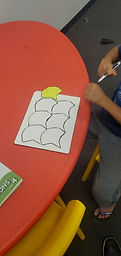Mr. Jake

Target 1​

Lesson Type:

Continuation

Geometry

:

Modeling

Visualize spatial relationships between shapes and planar space.

1:

Use reflectional and rotational symmetry to identify and describe tessellations.

2:

Understand that a tessellation is a repeated geometric figure that covers a plane without gaps or overlaps.

3:

Construct a tessellation and use it to create a pattern in which there are no empty spaces.

5th

Vocabulary:

Tesselation, Repeat

Activities:

Used a sticky note, scissors, and tape to make a shape to tesselate.Home Exploration

Guiding Questions:Absent Students:

Target 2

:

1:

Understand equivalent measurements.

2:

Understand how to convert from one unit to another.

3:

Use scale to reproduce a figure at either a bigger or smaller size (relate the measurement of the original figure to the new figure).

6th

Vocabulary:

Measurement, Convert, Unit

Activities:

Used a sticky note, scissors, and tape to make a shape to tesselate.

Converted the units of measurments to answer problems

Answered word problems that iUsed a sticky note, scissors, and tape to make a shape to tesselate.

Converted the units of measurments to answer problems

Answered word problems that invUsed a sticky note, scissors, and tape to make a shape to tesselate.

Converted the units of measurments to answer problems

Answered word problems that involve unit conversionUsed a sticky note, scissors, and tape to make a shape to tesselate.

Converted the units of measurments to answer problems

Answered word problems that involve unit conversiConverted the units of measurments to answer problems

Answered word problems that involve unit conversionHome Exploration

Guiding Questions:Target 3

:

Vocabulary:

Activities:Home Exploration